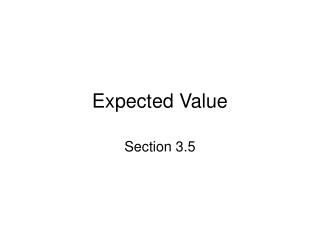# Expected Value - PowerPoint PPT PresentationDownload PresentationExpected Value

Expected Value
Download Presentation## Expected Value

- - - - - - - - - - - - - - - - - - - - - - - - - - - E N D - - - - - - - - - - - - - - - - - - - - - - - - - - -
##### Presentation Transcript

1. Expected Value Section 3.5

2. Definition • Let’s say that a game gives payoffs a1, a2,…, an with probabilities p1, p2,… pn. The expected value ( or expectation) E of this game is E = a1p1 + a2p2 + … + anpn. • Think of expected value as a long term average.

3. American Roulette • At a roulette table in Las Vegas, you will find the following numbers 1 – 36, 0, 00. There are 38 total numbers. • Let’s say we play our favorite number, 7. • We place a \$1 chip on 7. If the ball lands in the 7 slot we win \$35 (net winnings). If the ball lands on any other number we lose our \$1 chip. • What is the expectation of this bet? • To answer this question we need to know the probability of winning and losing. • The probability of winning is 1/38. The probability of losing is 37/38. • So the expectation is E = \$35(1/38) + (-\$1)(37/38) = (35-37)/38 = -2/38 = -\$0.053 • What this tells us is that over a long time for every \$1 we bet we will lose \$0.053. • This is an example of a game with a negative expectation. One should not play games when the expectation is negative.

4. Example 2 • On the basis of previous experience a librarian knows that the number of books checked out by a person visiting the library has the following probabilities: • Find the expected number of books checked out • by a person. • E = 0(0.15)+1(0.35)+2(0.25)+3(0.15)+4(0.05)+5(0.05) • E = 0 + 0.35 + 0.50 + 0.45 + 0.20 + 0.25 • E = 1.75

5. Two dice are rolled • A player gets \$5 if the two dice show the same number, or if the numbers on the dice are different then the player pays \$1. • What is the expected value of this game? • What is the probability of winning \$5? ANSWER 6/36 = 1/6. • What is the probability of paying a \$1? ANSWER 5/6. • Thus E = \$5(1/6) + (-\$1)(5/6) = 5/6 – 5/6 = 0. • The Expectation is \$0. This would be a fair game.

6. Who Wants to be a Millionaire? • Recall the game show Who Wants to be a Millionaire? Hosted by Regis Philbin. • Let’s say you’re at the \$125,000 question with no life-lines. The question you get is the following • What Philadelphia Eagles head coach has the most victories in franchise history? A. Earle “Greasy” Neale B. Buddy Ryan C. Dick Vermeil D. Andy Reid

7. More Millionaire • If you get the question right you will be at \$125,000. • If you get the question wrong you fall back to \$32,000. • Your third option is to walk away with \$64,000. • What to do, what to do? • Let’s do a mathematical analysis.

8. Mathematical analysis • What is the probability of guessing correctly? ANSWER ¼. • What is the probability of guessing incorrectly? ANSWER ¾. • What is our expectation? ANSWER E = \$125,000(1/4)+\$32,000(3/4) = \$55,250. This is less than the \$64,000 walk away value. • Decision: We should walk away.

9. What if we had a 50/50 • Then 2 of the choices would vanish. • Now the choices left will be A. Greasy Neale and D. Andy Reid. • Now E = (1/2)\$125,000 + (1/2)\$32,000 = \$78,500 > \$64,000. • We should give it a shot. • The answer is A. Greasy Neale (for now, later this season Andy Reid will pass Neale).